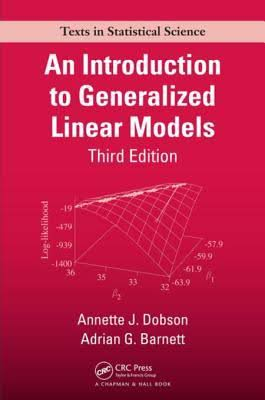An Introduction to Generalized Linear Models

I recently had a chunk of leave, and I thought that a good use of my time would be to read “An Introduction to Generalized Linear Models”, by Annette J. Dobson and Adrian G. Barnett (2008). My statistical background is somewhat haphazard, so this book really filled in some of the cracks in my foundation. It provides an overview of the theory, illustrated with examples, and includes code to implement the methods in both R and Stata. The book covers a wide range of applications, and every chapter ends with exercises to help cement the knowledge gained.book cover

Before getting into the topic of generalized linear models itself, the book starts with two introductory chapters, which provide some statistical background that is necessary for the rest of the book. I found the second chapter, on the process of statistical modelling, particularly helpful, as a summary of what we were trying to achieve. Building statistical models is only one step in a long process that starts with graphical exploratory analysis and understanding variables, proceeds through describing various candidate models and estimating their unknown parameters, and finally checking the validity of each model. It helped me to keep that bigger picture in mind while focusing on each particular step.

Chapter 3 introduces generalized linear models themselves. These are models where the response variable is expected to be related (possibly via some link function) to a linear combination of a number of explanatory variables, but with some distribution around that expected value. The best known distribution is, of course, the normal distribution, but any distribution in the so-called ‘exponential family’ can be used to describe the variation of the response variable. The book further expands on some of the distributions and their particular uses, although not before spending two chapters describing the frequentist bread-and-butter that is estimation and inference.

Normal models are good for continuous responses, such as the growth of a plant under different circumstances, while logistic models would be used for finite responses such as ‘dead’ or ‘alive’ (nominal), or ‘poor’, ‘average’, or ‘excellent’ (ordinal). Poisson models are the prefered distributional choice where the response is count data, such as in a contingency table. Each of these scenarios was explored with detailed examples and sample code. Having these cases presented one after each other was a useful way to to see what was common to all methods, and also how each case was different.

The next two chapters are dedicated to two special cases. The first is ‘survival analysis’, where the problem of censored data (people surviving beyond the duration of the study) is addressed. The second looks at correlated data, where data is collected from the same person over time (longitudinal data) or from groups of similar subjects (panel data). These two chapters give some insight into the problems that could occur if models are built without due consideration of the subtleties of the situation.

To complete the book, the last three chapters describe the Bayesian paradigm for the methods that were described up to that point with a frequentist approach. The first is a chapter introducing Bayesian analysis, followed by a description of Markov Chains, and the Monte Carlo Method of numerical integration. The book concludes with a chapter where previous examples are repeated using Bayesian models, which includes code for implementing them in the WinBUGS program. As computing power is increasing, the Bayesian paradigm is becoming more and more important. With this in mind, it was valuable to see how the two different paradigms compare, both in the way that the models are set up, and in their results.

Overall, I learnt a lot from reading this book. I have a much clearer idea of how the different techniques relate to each other, and how the general processes of model fitting and checking can be performed in different circumstances. And with R’s glm() function doing most of the legwork, I can be more confident in applying these methods in my work.